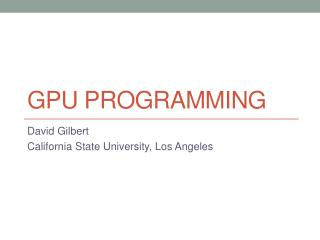DownloadDownload PresentationGPU Programming

# GPU Programming

Télécharger la présentation## GPU Programming

- - - - - - - - - - - - - - - - - - - - - - - - - - - E N D - - - - - - - - - - - - - - - - - - - - - - - - - - -
##### Presentation Transcript

1. GPU Programming David Gilbert California State University, Los Angeles

2. Outline • CUDA • CPU vs GPU Architecture • Scalability • Blocks • Performance • Speed Up • Graphics Cards • How It Works • Program Flow • When to Use the GPU • Example: Matrix Row Sum • References

3. CUDA • Compute Unified Device Architecture (CUDA) • High performance computing on your GPU • CUDA is a proprietary architecture for GPU Computing, there is also OpenCL which runs on AMD/ATI

4. CPU vs GPU Architecture • ALU does the computations

5. Scalability • Code automatically scales upward • GPUs with more cores will execute the same code in less time • Can add additional graphics cards to your computer and gain exponential performance increases!

6. Blocks • Essentially Groups • Block Size and ThreadsPerBlock are defined before the memory is copied to the graphics card. • To access a thread in ablocki = blockIdx.x + threadIdx.x;j = blockIdx.y + threadIdx.y;

7. Performance • Super computer performance is measured in Floating Point Operations Per Second (FLOPS) • Megaflops = 10^6 • Gigaflops = 10^9 • Teraflops = 10^12 • Petaflops = 10^15 • Japan’s K Computer • 10.51 Petaflops • Nvidia GTX 480 • ~1300 gigaflops • Core i7 920 @3.4Ghz • 69 gigaflops

8. Graphics Cards • Consumer • AMD 6950, \$250 • 2.25 TFLOPs Single Precision compute power • 562.5 GFLOPs Double Precision compute power • 1408 Stream Processors • Nvidia GTX 470, \$150 • 1.09 TFLOPs Single Precision compute power • 544.32 GFLOPs Double Precision compute power • 448 Cuda Cores • About \$1 per TFLOP

9. Speed Up?

10. How it works • Computer dumps the load onto the GPU • GPU does the computing • GPU returns the results to System Memory • This transfer is the biggest bottleneck in the system Code CPU GPU Results

11. Program Flow • Allocate System Memory • Allocate Device Memory • Copy Memory from System to Device • Execute the Code • Copy Results back to the System from the Device • Free Device Memory • Process Results • Free System Memory • Lines 3 and 5 create the bottleneck

12. When to Use the GPU • Let dT = transfer time between device and system • Let st = serial execution time • Let pt = parallel execution time 2(dT) + pt < st

13. Example: Matrix Row Sum Block size, 4X1

14. Example: Matrix Row Sum // Device code __global__ void RowSum(float* B, float* Sum, intN, int M) { inti = blockDim.x * blockIdx.x + threadIdx.x;int j = blockDim.y * blockIdx.y + threadIdx.y; if (i < N && j < M) C[j] += B[i][j];} • B is the matrix being summed • Sum is the array storing the row sum • N is # of rows • M is # of cols

15. Example: Matrix Row Sum int main(){ int M = 4, N = 4; // Allocate System Memory size_t size = N*M*sizeof(float); float * h_B = (float *)malloc(size); float * h_sum = (float *)malloc(size); // Allocate Device Memory float * d_B, * d_sum; cudaMalloc(&d_B, size); cudaMalloc(&d_sum, size); // Copy System Memory to Device cudaMemcpy(d_B, h_B, size, cudaMemcpyDeviceToHost); // Execute the code intthreadsPerBlock = 4; intblocksPerGrid = 4; RowSum<<<blocksPerGrid, threadsPerBlock>>>(d_B, d_sum, N, M); // Copy Results from Device Back to System Memory cudaMemcpy(h_sum, d_sum, size, cudaMemcpyDeviceToHost); // Free device Memory cudaFree(d_B); cudaFree(d_sum); // Process Results print results… // some method to display results // Free System Memory free(h_B); free(h_sum); return 0; }

16. Example: Matrix Row Sum • Now, imagine a matrix of 1000 x 1000 • I don’t guarantee that this code will run